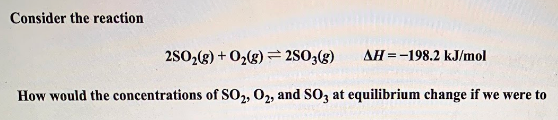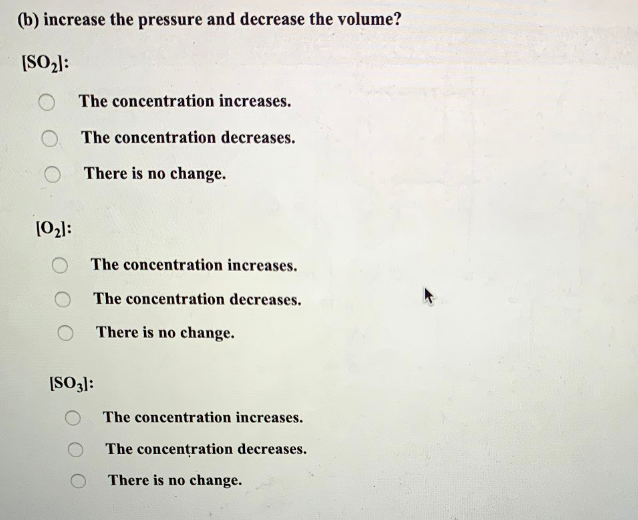# Consider the reaction 2SO2(g) + O2(g) ⇌ 2SO3(g) ΔH = -198.2 kJ/mol How would the concentrations of SO2, O2, and SO3 at equilibrium change if we were to (b) increase the pressure and decrease the volume? [SO2]: a) The concentration increases. b) The concentration decreases. c) There is no change [O2]: a) The concentration increases. b) The concentration decreases. c) There is no change [SO3]: a) The concentration increases. b) The concentration decreases. c) There is no change